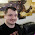Thursday, December 13, 2012

Random Dungeon Generation

For all of you who have ever wanted to do something like this but couldn't figure out what exactly that algorithm is trying to tell you to do: Here is the easiest way to generate a random (and ok looking) dungeon.

Note that this algorithm doesn't care if it cuts through another room to get to the room it's trying to connect to. To fix this, you can use the A* algorithm to go around certain rooms when placing hallways.

Also, there are two issues that you should keep in mind.

Firstly, it is possible for the while loop to go into an infinite loop for certain map sizes (or bad luck when placing rooms). One way to get around this might be to have a counter run in the while loop and exit the loop when the counter reaches some number.

Secondly, it is possible for some rooms to remain separate from all other rooms. This will be the case when two rooms connect to each-other and are too far away from any other rooms to have corridors pass through them. For simplicity, I've chosen to not factor that scenario into the algorithm.

Scroll down to see the algorithm

//Create Data Structure
map = new array[width][height]
for each value in the 2d array
map[i][j] = empty_cell

//How many rooms?
minimum_rooms = (width * height) / 300
maximum_rooms = (width * height) / 150
room_count = random(minimum_rooms, maximum_rooms)

//Room sizes?
width_root = square_root(width * 2)
height_root = square_root(height * 2)
minimum_width = (width * 0.5) / width_root
maximum_width = (width * 2.0) / width_root
minimum_height = (height * 0.5) / height_root
maximum_height = (height * 2.0) / height_root

//Create rooms
roomList = new list
for i = 0 to room_count
{
ok = false
//This while loop runs until we find somewhere the room fits
//There are faster ways of doing this but for the map sizes I'm
//using, it serves my needs and is fast enough.
while (!ok)
{
room.x = random(0, width)
room.y = random(0, height)
room.w = random(minimum_width, maximum_width)
room.h = random(minimum_height, maximum_height)

if (room outside bounds of map)

continue

if (room overlaps with other room)

continue

//If we reach this point, the room can be placed
ok = true
}
}

//Connect Rooms
connectionCount = roomCount
connectedCells = new list
for i = 0 to connectionCount
{
roomA = roomList[i]
roomB = random other room to connect to

//Increasing this number will make hallways straighter
//Decreasing this number will make halways skewer
sidestepChance = 10
pointA = random point in roomA
pointB = random point in roomB

//This is a type of drunken/lazy walk algorithm
while (pointB != pointA)
{
num = random(0, 100)
if (num < sidestepChance)
{
if (pointB.x != pointA.x)
increase/decrease pointB.x depending on where pointA.x is
}

if (we didn't change the x position)

{
if (pointB.y != pointA.y)
increase/decrease pointB.y depending on where pointA.y is
}
}
}

//Create Map Data
for i = 0 to roomCount
{
for every cell in a room
map[x][y] = room_cell
}

for i = 0 to connectedCells

{
for every cell where we put a connector earlier
map[x][y] = hallway_cell
}

//Lastly, create walls using tessellation
for every cell that is empty but touches a room or corridor cell
map[x][y] = wall_cell

1.Great algorithm! Thanks for showing us the way!

2.Thanks for this. This has definitely earned a place in my code snippet library!!

3.Pretty cool, made a js version using your algorithm here http://jsfiddle.net/loktar/Y9VtP/embedded/result/ makes some neat little dungeons. Although I used hard coded values for the room amount, and room sizes.

4.The text kerning makes me sad.

5.lol yeah makes it look like Im some sort of idiot spammer.

6.Awesome! Thanks for sharing :)

7....But now can it have multiple stories?

8.This is a brilliantly simple algorithm, and it's fairly straightforward to convert it into any coding language. I'm now using it as a base for my own dungeon generation; it's even been given its own class library for use in future projects! Thanks for sharing it with the world :)

9.Is there a reason for using the square root of map sizes in finding out your room dimensions? Or is it just flair?

1.That part was found largely through trial and error. It's a nice way to calculate the width and height root variables that I then employ in the calculation of the min and max sizes of rooms.